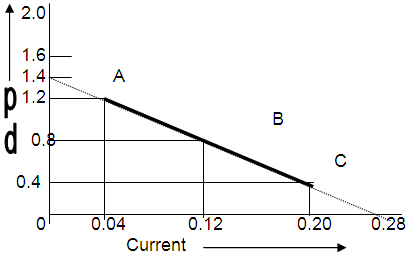# Potential difference across terminals of a cell were measured (in volts) against different currents ( in ampere) flowing through the cell. A graph was drawn which has a straight line ABC.Using the data given in the graph determine,(I) the emf and(ii) the internal resistance of the cell.emf=1.4

v=e-ir ==> r=(e-v)/i=(1.4-1.2)/0.04=5

• -17
Plz thumbs up• 54
Find emf and internal resistance• -9
Find EMF and internal resistance of a cell• 1